Maths
Study Material

# Sarrus Rule for Calculating Determinants of Matrices of Order 3

This blog contains how the Sarrus rule can be used to find out the determinant of any matrix of order 3 within seconds.

4 minutes long
Posted by Tanwir Silar, 1/9/2021Hesap Oluştur

Got stuck on homework? Get your step-by-step solutions from real tutors in minutes! 24/7. Unlimited.

Have you ever wondered if there is a way to easily calculate the determinant of a matrix of order 3 without going through the tedious process of calculating the minors. If yes, then you are at the right place.
Without any delay, lets look at the Sarrus rule.

## What does the Sarrus rule say?

It says that the determinant can be obtained by the algebraic sum of a series of multiplications when the given 3×3 matrix is written in the following form.As shown in the figure above, we need to multiply the elements along the arrows and then add the resultant product if it originated from a red arrow or subtract it if originated from a blue arrow.
If the above procedure is followed, then the determinant is equals to a11a22a33 + a12a23a31 + a13a21a32 – a31a22a13 – a32a23a11 – a33a21a12. This is Sarrus rule.

## Comparing the methods of finding determinant

Let A =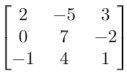, let us find the determinant by expanding along first row and also using Sarrus rule.

#### Applying Sarrus rule: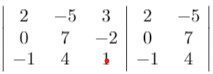det(A) = 2*7*1 + (-5)*(-2)*(-1) + 3*0*4 – (-1)*7*3 – 4*(-2)*2 – 1*0*(-5)
= 14 – 10 + 0 + 21 + 16 – 0
= 41

#### Expanding along first row:

The first row has the elements 2, -5, 3.
The minor of 2 is =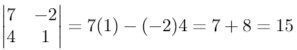The minor of -5 is =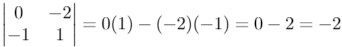The minor of 3 is =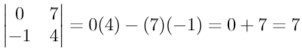After finding the minors, to calculate the determinant, the formula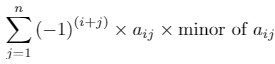is used.
We get,
det(A) is equals to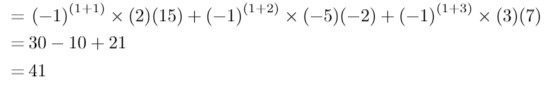The above comparison proves the easiness associated with Sarrus rule. So, the next time you come across the task of finding the determinant of a 3×3 matrix, fret not, as you have the Sarrus rule in your arsenal.